# 浅谈算法和数据结构三 合并排序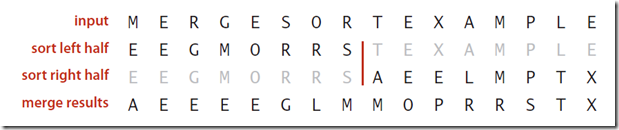# 一 原理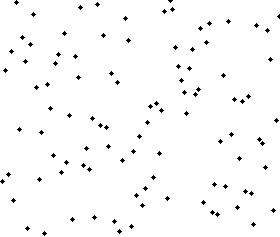1. 申请空间，使其大小为两个已经排序序列之和，然后将待排序数组复制到该数组中。
2. 设定两个指针，最初位置分别为两个已经排序序列的起始位置
3. 比较复制数组中两个指针所指向的元素，选择相对小的元素放入到原始待排序数组中，并移动指针到下一位置
4. 重复步骤3直到某一指针达到序列尾
5. 将另一序列剩下的所有元素直接复制到原始数组末尾

``````private static void Merge(T[] array, int lo, int mid, int hi)
{
int i = lo, j = mid + 1;
//把元素拷贝到辅助数组中
for (int k = lo; k <= hi; k++)
{
aux[k] = array[k];
}
//然后按照规则将数据从辅助数组中拷贝回原始的array中
for (int k = lo; k <= hi; k++)
{
//如果左边元素没了， 直接将右边的剩余元素都合并到到原数组中
if (i > mid)
{
array[k] = aux[j++];
}//如果右边元素没有了，直接将所有左边剩余元素都合并到原数组中
else if (j > hi)
{
array[k] = aux[i++];
}//如果左边右边小，则将左边的元素拷贝到原数组中
else if (aux[i].CompareTo(aux[j]) < 0)
{
array[k] = aux[i++];
}
else
{
array[k] = aux[j++];
}
}
}``````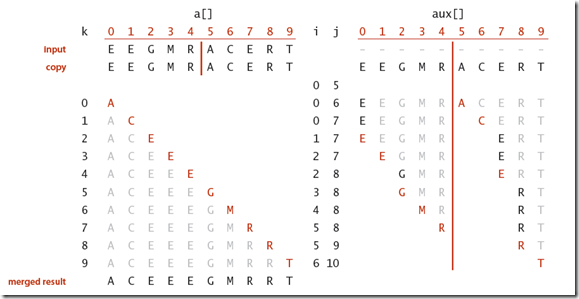# 二 实现

``````public class MergeSort<T> where T : IComparable<T>
{
private static T[] aux; // 用于排序的辅助数组
public static void Sort(T[] array)
{
aux = new T[array.Length]; // 仅分配一次
Sort(array, 0, array.Length - 1);
}
private static void Sort(T[] array, int lo, int hi)
{
if (lo >= hi) return; //如果下标大于上标，则返回
int mid = lo + (hi - lo) / 2;//平分数组
Sort(array, lo, mid);//循环对左侧元素排序
Sort(array, mid + 1, hi);//循环对右侧元素排序
Merge(array, lo, mid, hi);//对左右排好的序列进行合并
}
...
}``````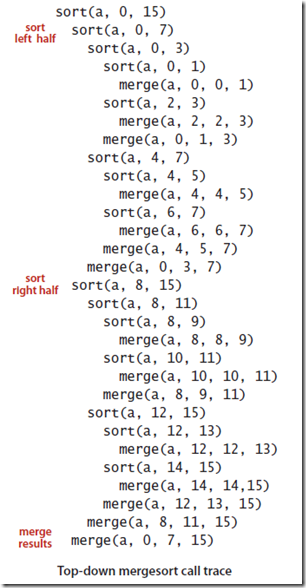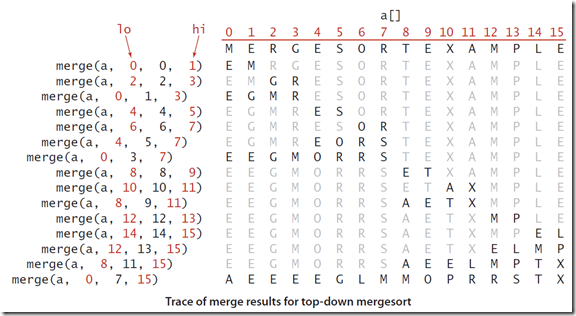# 三 图示及动画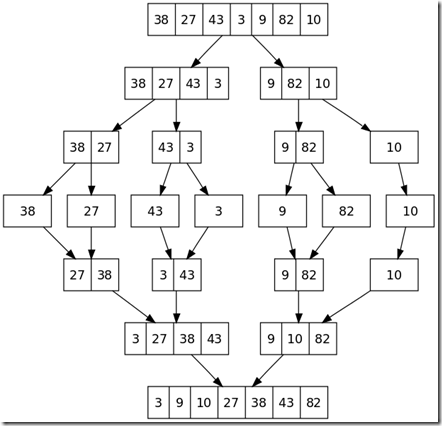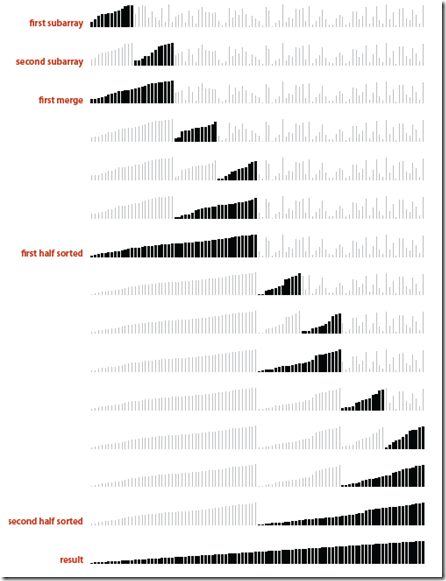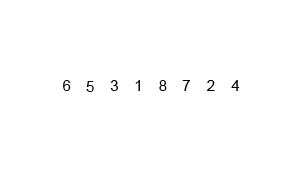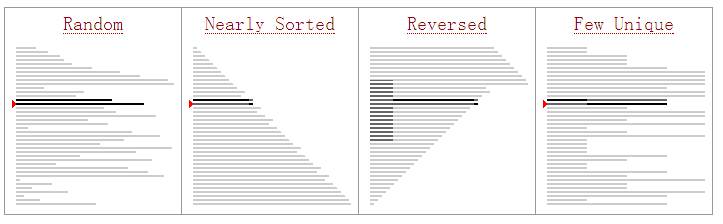# 四 分析

1. 合并排序的平均时间复杂度为O(nlgn)

D(N)=2D(N/2)+N,N>1;

D(N)=0,N=1; (当N=1时，数组只有1个元素，已排好序，时间为0)

Prof1：处于方便性考虑，我们假设数组N为2的整数幂，这样根据递归式我们可以画出一棵树：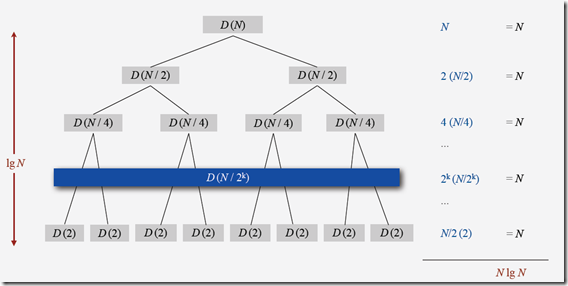​ D(N)=(lgN+1)N=NlgN+N=NlgN

Prof2：我们在为递归表达式求解的时候，还有一种常用的方法就是数学归纳法，

1. 当N=1 时，D(1)=0,满足初始条件。
2. 为便于推导，假设N是2的整数次幂N=2k, 即D(2k)=2klg2k = k*2k
3. 在N+1 的情况下D(N+1)=D(2k+1)=2k+1lg2k+1=(k+1) * 2k+1,所以假设成立，D(N)=NlgN.

2. 合并排序需要额外的长度为N的辅助空间来完成排序

# 五 几点改进

1. 当划分到较小的子序列时，通常可以使用插入排序替代合并排序

``````private const int CUTOFF = 7;//采用插入排序的阈值
private static void Sort(T[] array, int lo, int hi)
{
if (lo >= hi) return; //如果下标大于上标，则返回
if (hi <= lo + CUTOFF - 1) Sort<T>.SelectionSort(array, lo, hi);
int mid = lo + (hi - lo) / 2;//平分数组
Sort(array, lo, mid);//循环对左侧元素排序
Sort(array, mid + 1, hi);//循环对右侧元素排序
Merge(array, lo, mid, hi);//对左右排好的序列进行合并
}``````

2. 如果已经排好序了就不用合并了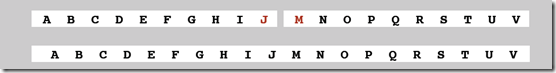``````private static void Sort(T[] array, int lo, int hi)
{
if (lo >= hi) return; //如果下标大于上标，则返回
if (hi <= lo + CUTOFF - 1) Sort<T>.SelectionSort(array, lo, hi);
int mid = lo + (hi - lo) / 2;//平分数组
Sort(array, lo, mid);//循环对左侧元素排序
Sort(array, mid + 1, hi);//循环对右侧元素排序
if (array[mid].CompareTo(array[mid + 1]) <= 0) return;
Merge(array, lo, mid, hi);//对左右排好的序列进行合并
}``````

3. 并行化

# 七 结语

Do you have any suggestions for improvement?